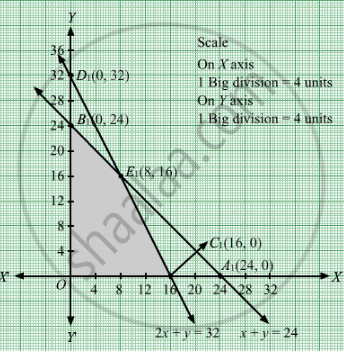# A Small Firm Manufacturers Items a and B. the Total Number of Items a and B that It Can Manufacture in a Day is at the Most 24. - Mathematics

Sum

A small firm manufacturers items A and B. The total number of items A and B that it can manufacture in a day is at the most 24. Item A takes one hour to make while item B takes only half an hour. The maximum time available per day is 16 hours. If the profit on one unit of item A be Rs 300 and one unit of item B be Rs 160, how many of each type of item be produced to maximize the profit? Solve the problem graphically.

#### Solution

Let the firm manufacture items of A and items of B per day.
Number of items cannot be negative.
Therefore, x ≥0 and y ≥0.

It is given that the total number of items manufactured per day is at most 24.

∴ x + y ≤ 24

Item A takes 1 hour to make and item B takes 0.5 hour to make.

The maximum number of hours available per day is 16 hours.

∴ x + 0.5y ≤ 16

If the profit on one unit of item A be Rs 300 and one unit of item B be Rs 160.Therefore, profit gained on items of A and items of B is
Rs 300x and Rs 160y respectively.

∴ Total profit, Z = 300x + 160y

Thus, the mathematical formulation of the given problem is:

Maximise Z = 300x + 160y

subject to the constraints

xy ≤ 24

x + 0.5y ≤ 16

xy ≥0

First we will convert inequations into equations as follows:
x + y = 24, x + 0.5y = 16, x = 0 and y = 0

Region represented by x + y ≤ 24:
The line x + y = 24 meets the coordinate axes at A1(24, 0) and B1(0, 24) respectively. By joining these points we obtain the line x + y = 24. Clearly (0,0) satisfies the x + y = 24. So, the region which contains the origin represents the solution set of the inequation
x + y ≤ 24.

Region represented by x + 0.5y ≤ 16:
The line x + 0.5y = 16 meets the coordinate axes at C1(16, 0) and D1(0, 32) respectively. By joining these points we obtain the line x + 0.5y = 16. Clearly (0,0) satisfies the inequation x + 0.5y ≤ 16. So,the region which contains the origin represents the solution set of the inequation x + 0.5y ≤ 16.

Region represented by ≥ 0 and y ≥ 0:
Since, every point in the first quadrant satisfies these inequations. So, the first quadrant is the region represented by the inequations x ≥ 0, and y ≥ 0.

The feasible region determined by the system of constraints x + y ≤ 24, x + 0.5y ≤ 16, x ≥ 0and y ≥ 0 are as follows.The corner points are O(0, 0) ,C1(16, 0), E1(8, 16) and B1(0, 24).

The value of Z at these corner points are as follows:

 Corner point Z = 300x + 160y O(0, 0) 0 C1(16, 0) 4800 E1(8, 16) 4960 B1(0, 24) 3840

Thus, the maximum value of Z is 4960 at E1(8, 16).

Thus, 8 units of item A and 16 units of item B should be manufactured per day to maximise the profits.

Concept: Graphical Method of Solving Linear Programming Problems
Is there an error in this question or solution?

#### APPEARS IN

RD Sharma Class 12 Maths
Chapter 30 Linear programming
Exercise 30.4 | Q 29 | Page 54